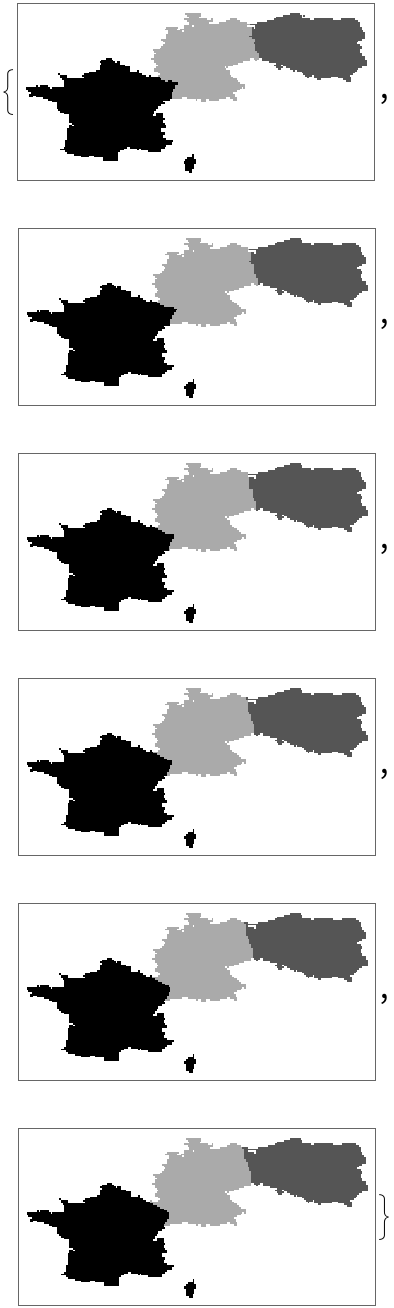#Function Repository Resource:

# Cartogram

Generate geometrically distorted maps, where the distortion conveys information derived from the input data

Contributed by: Richard Lapin
 ResourceFunction["Cartogram"][reg] generates a plot of width 100 in which geographic region reg specified in the form of an Entity object are displayed after 30 iterations; the destortion is based on equalising the population densities by default. ResourceFunction["Cartogram"][{reg1 → val1, reg2 → val2,…}] generates a plot in which geographic regions reg1 and reg2 specified in the form of Entity objects are displayed after 30 iterations, where the distortion is based on the values val1 and val2. ResourceFunction["Cartogram"][reg,iterations] generates a plot in which geographic region reg is displayed after the specified number of iterations. ResourceFunction["Cartogram"][reg,iterations,res] generates a plot of width res. ResourceFunction["Cartogram"][reg,iterations,res,plotAll] generates a list of plots of width res from the undistorted map to the specified number of iterations.

## Details and Options

The reg can be Entity objects such as countries corresponding to extended geographic regions, or geometric constructs with GeoPosition coordinates.
The val is a number.

## Examples

### Basic Examples

A list of different examples:

 In:=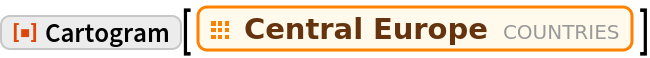Out=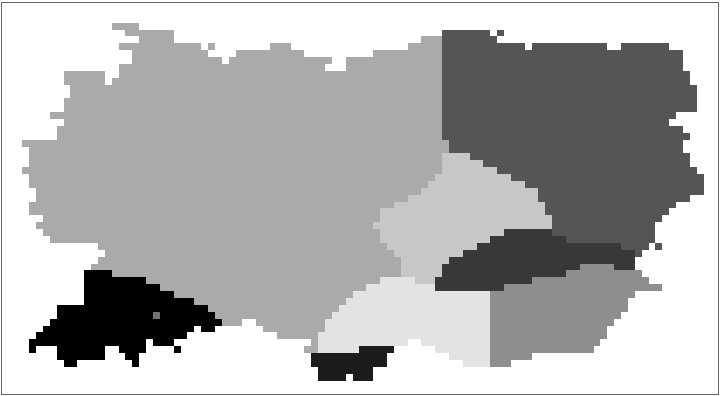Running the function for a list of countries:

 In:=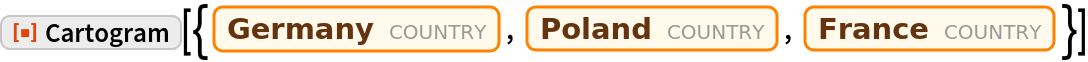Out=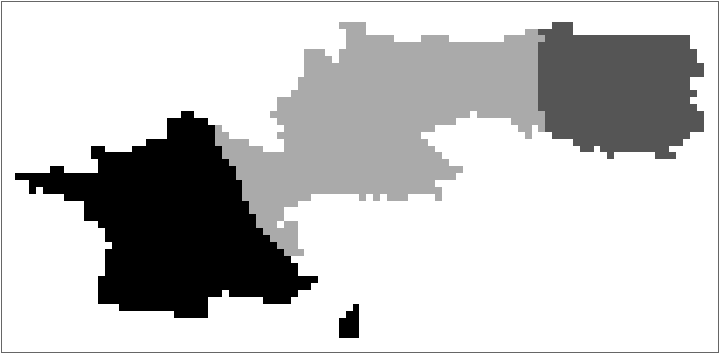Running the function for a list of countries using the user-input values:

 In:=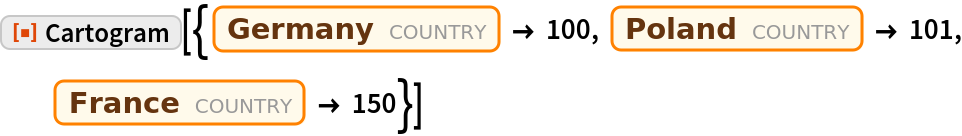Out=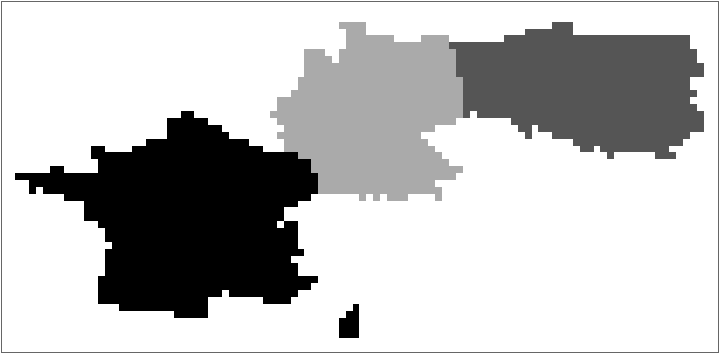Running the function for just one iteration:

 In:=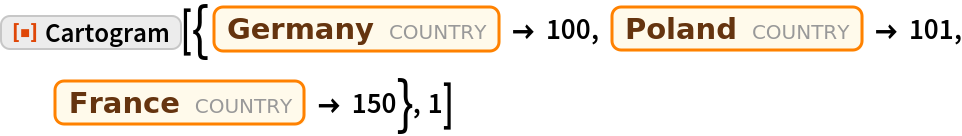Out=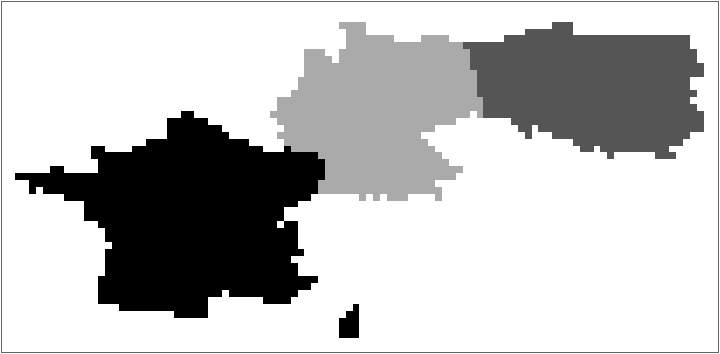Running the function for 5 iterations with the width of 400 pixels.

 In:=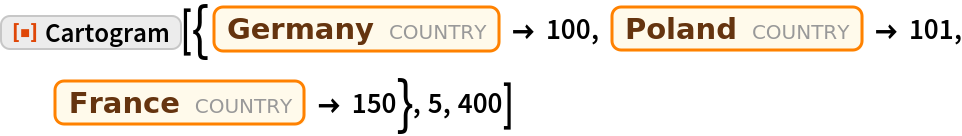Out=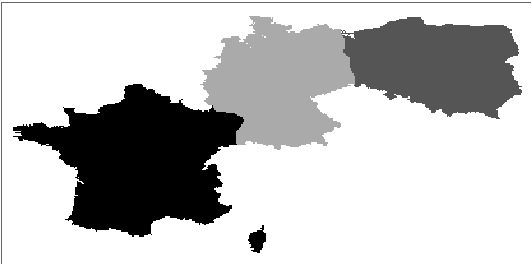Returns all steps of first 5 iterations:

 In:=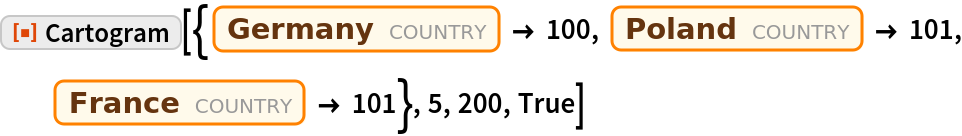Out=